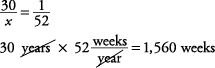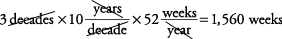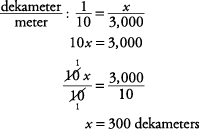## Converting Units of Measure

If 36 inches equal 1 yard, 3 yards equal how many inches?

Intuitively, 3 × 36 = 108 in

By proportion, using yards over inches,Remember: Place the same units across from each other—for example, inches across from inches. Then solve.Cross multiply.

x = 108 in

If 2.2 pounds equal 1 kilogram, 10 pounds equal approximately how many kilograms?

Intuitively, 10 ÷ 2.2 ≈ 4.5 kg

By proportion, using kilograms over pounds,Cross multiply.

2.2 x = 10

Divide and round off to the nearest tenth.1 year equals 52 weeks, soTherefore, there are 1,560 weeks in 3 decades.

Notice that this was converted step by step. It can be done in one step, as follows:3 × 10 × 52 = 1,560 weeks.

If 1,760 yards equal 1 mile, how many yards are in 5 miles?Therefore, there are 8,800 yards in 5 miles.

If 1 kilometer equals 1,000 meters, and 1 dekameter equals 10 meters, how many dekameters are in 3 kilometers?

Since there are 1,000 meters in one kilometer, there will be 3,000 meters in 3 kilometers. Now use a proportion that compares dekameters with metersTherefore, there are 300 dekameters in 3 kilometers.